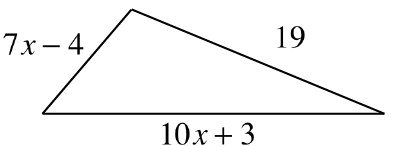### Home > GC > Chapter 1 > Lesson 1.2.2 > Problem1-65

1-65.The perimeter (the sum of the side lengths) of the triangle at right is $52$ units. Write and solve an equation based on the information in the diagram.  Use your solution for $x$ to find the measures of each side of the triangle.  Be sure to confirm that your answer is correct.

Find the sum of the side lengths.

The sum of the side lengths is the perimeter, which we know from the problem is $52$ units. Set the expression from Step 1 equal to $52$ and solve for $x$.

$(7x-4)+(19)+(10x+3)$

$\begin{array}{r} (7x-4)+(19)+(10x+3)=52\\ 17x+18=52 \end{array}$

Continue solving to find the value of $x$.

Determine each of the side lengths by substituting the value of $x$ into the expression given for each side.